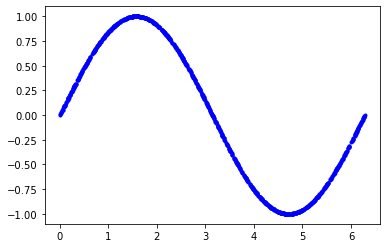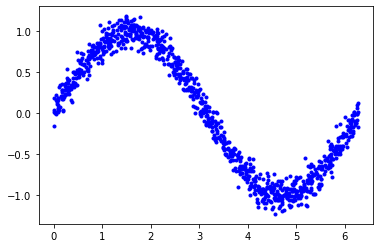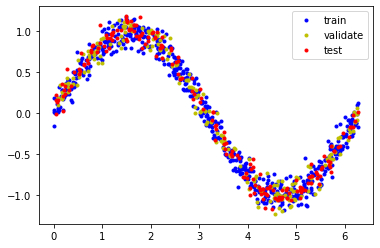In :
!date

Mon 01 Nov 2021 05:13:53 PM EDT

In :
import numpy as np
import tensorflow as tf

In :
import matplotlib.pyplot as plt

In :
import math

In :
# we'll generate this many sample datapoints
samples = 1000
seed = 1337
# seed value to get same random numbers each time we run notebook. any number can be used.

np.random.seed(seed) # sets np random seed which is stored in memory
tf.random.set_seed(seed) # sets tf random seed so it is stored in memory

x_values = np.random.uniform(low=0, high=2*math.pi, size=samples)
# populates x_values with a range of 0 to pi twice with a size of 1000
# which  means it's an array?
np.random. shuffle(x_values)
# shuffles value in container

y_values = np.sin(x_values)
# sets y_values to sin of the x values
plt.plot(x_values, y_values, 'b.')
plt.show()
# plotsIn :
type(x_values)
np.shape(x_values)

Out:
(1000,)
In :
y_values += 0.1 * np.random.randn(*y_values.shape)
# adds a wider variety of randomness to a nearly perfect line.

In :
plt.plot(x_values, y_values, 'b.')
plt.show()In :
# splitting data for testing and training

train_split = int(0.6 * samples)
# this takes 60% of data for training
test_split = int(0.2 * samples + train_split)
# this takes twenty percent of samples plus the train split which is 60
# don't understand the test split so much.

train_split
test_split

Out:
800

the splits are indicies, locations in where the list would be split into the next function

In :
x_train, x_validate, x_test = np.split(x_values, [train_split, test_split])
y_train, y_validate, y_test = np.split(y_values, [train_split, test_split])

In :
assert (x_train.size + x_validate.size + x_test.size) == samples

In :
# no return is good return on assert

In :
plt.plot(x_train, y_train, 'b.', label="train")
plt.plot(x_validate, y_validate, 'y.', label="validate")
plt.plot(x_test, y_test, 'r.', label="test")
plt.legend()
plt.show()This is something I somewhat understand. Testing and validation.

I was asked in an interview - how do you validate your data?

I literally had no clue. I mean, if I'm working with it, and it's been collected, cleaned, and now at my finger tips, isn't it valid? (Got too ethereal, way too quick)

I was way out of the ballpark to understand what the guy meant. It took me until a few days ago to understand what was meant by that question.

Part of the tools of machine learning is the data itself. Before we get away from ourselves, what was I working on above?

One of these other machine learning books I've purchased (totaling in three now, yes a lot has happened since the start of these blurbs), the author starts off with a pretty low level example on how to build a model to predict something that we can predict nowadays. The top most graph is a sine wave. The extent of what I know about sine waves is that they are wavey and that 'sine' is the 's' in soh cah toa which is a helpful mnemonic to help you remember something about math...which is...uh..well...moving forward.

The second graph is the visual result of splitting up the data after adding some "randomness" to it so that we can use the data that we have to build a model to make predictions about data we don't have.

In the code, it can be seen that I am "slicing" up parts of the data, taking 60% of the data for training the model (the data we'll use to teach our young machine to recognize and predict sine waves).

Then once the little machine is trained, we see if it can predict points from the test data.

Why do we split it that way?

I have no clue. But now I kind of understand what must be done, as a task, to begin validating data. That's one whole interview question!

What I realized in purchasing two more machine learning books -

I have lots of programming books and have even given some away as a result of the guilt I had about not finishing them. All my programming books not including the last three are in a wide variety of subjects. NLP, Data Visualization w/JS, Linux something or another but I don't have any that have any (or very little) cross over. In the little bit of studying I've been doing of the first book, Hands on ML and then after moving to the next ones, I'm starting to gain a better understanding of why all these ML books have such a concept heavy introduction.

It's because machine learning is complicated as heck and it comes with it's own language to understand it's main focus, the architecture of these models.

The two other books I purchased are tinyML and FastAI for coders. I am particularly excited about the tinyML one because there is follow-alongs for running machine learning programs on a small ARM computer (a 15 dollar computer) and the FastAI for coders features an entire online shmorgasboard of free resources that go with the book. They're free to you too.

That's really it. No justification for spending an inordinate amount of money on books.

Not yet anyway.